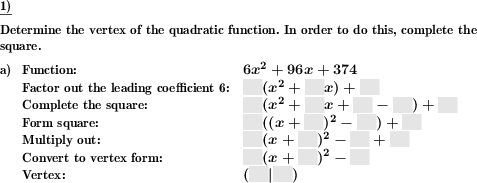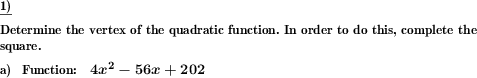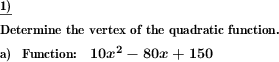Custom math worksheets at your fingertips# Details for problem "Quadratic formula: Complete square to determine vertex"

Quickname: 9004

Junior High School, High School, Senior High School.

## Summary

To determine the vertex of a square function, convert it to vertex form by completing the square.

## Examples## Description

The vertex of a quadratic formula is to be determined by transforming the function into vertex form. To do this, complete the square.

If desired, the solution is described in the solution sheet in the steps

1. Factor out the guide coefficient
2. Complete the square
3. Introduce the square
4. Multiply out
5. Write the quadratic function in vertex form
6. State the vertex

is presented in detail. These steps can be presented in the problem statement as cloze text, the correct values must then be entered.

In the problem definition the vertex of a quadratic function is asked for. It can be set whether the solution path via the quadratic addition should also be indicated.

For simplification, the problem can be set so that the guide coefficient, i.e. the factor for x squared, is always one.

The number of problems can also be set.

Download free printable worksheets for this math problem here. The worksheet contains the problems only, the solution sheet includes the answers. Just click on the respective link.

•Worksheet 1Solution sheet with answers
•Worksheet 2Solution sheet with answers
•Worksheet 3Solution sheet with answers

If you can not see the solution sheets for download, they may be filtered out by an ad blocker that you may have installed. If this is the case, please allow ads for this page and reload the page. The solution sheets will then reappear.

• Do these sample worksheets do not really fit?
• Do you need more math worksheets, with a different level of difficulty?
• Would you like to combine different problems on a worksheet and adjust them to your needs?
• As a teacher, you can put together your own worksheets using the automatically generated math problems provided.
With a free initial credit, you can start creating your own math worksheets in a few minutes.

You can try it for free! Register here, to create custom worksheets now!

## Customization options for this problem

Parameter
Possible values
Number of problems
1, 2, 3, 4, 5, 6
Mention completion of square
Yes, No
Indicate steps
no, with solution, with problem&solution
Yes, No

## Similar problems

Remark
Description
Complete the square to solve the equation
To solve a quadratic equation, complete the square.